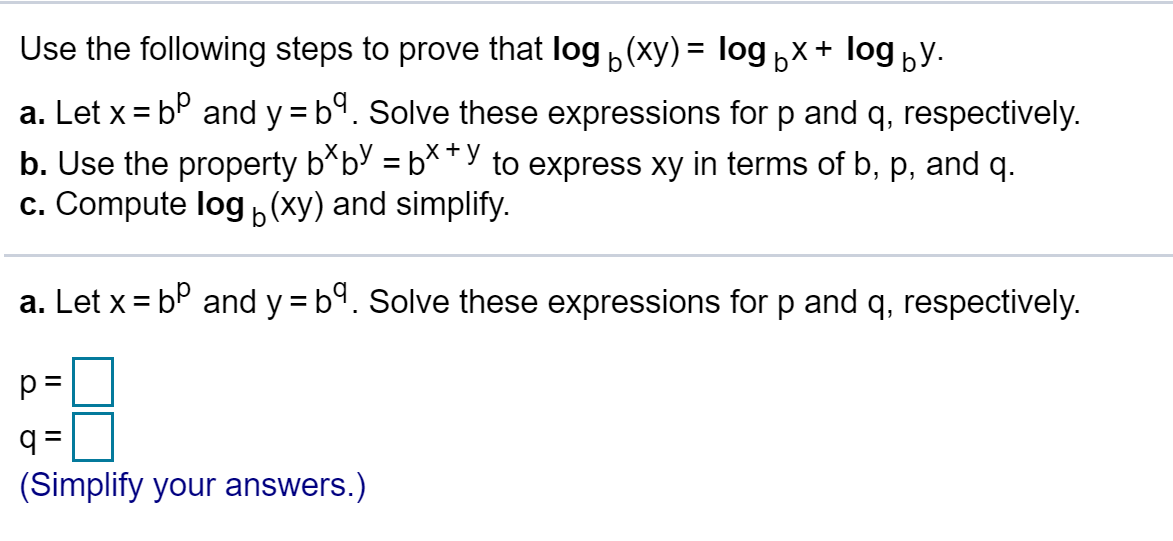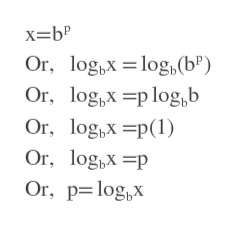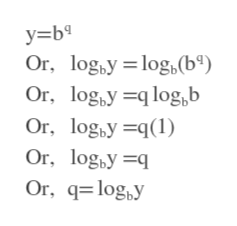Use the following steps to prove that log(xy)= logbx+ logby.a. Let x bP and y = b. Solve these expressions for p and q, respectively.b. Use the property b*bV = b**"y to express xy in terms of b, p, and q.c. Compute log (xy) and simplify.+ba. Let x bP and y b. Solve these expressions for p and q, respectively.q =(Simplify your answers.)

Questionhelp_outlineImage TranscriptioncloseUse the following steps to prove that log(xy)= logbx+ logby. a. Let x bP and y = b. Solve these expressions for p and q, respectively. b. Use the property b*bV = b**"y to express xy in terms of b, p, and q. c. Compute log (xy) and simplify. + b a. Let x bP and y b. Solve these expressions for p and q, respectively. q = (Simplify your answers.) fullscreen
Step 1

We take log base b on both sides of x=b^p.

p=log_b (x)help_outlineImage Transcriptionclosex=b" Or, log,Xlog,(b") Or, og,plog,b = Or, og,p Or, logx p Or, p logx fullscreen
Step 2

We take log base b on both sides of y=b^q.

Answer(a): p=log base b (x)

q=log base b (y)help_outlineImage Transcriptionclosey=b Or, logby log (b°) Or, logy log,b Or, logyq1 Or, log,y q Or, qlogy fullscreen
Step 3

We know x=b^p and y=b^q.

So, xy=b^(p...

Want to see the full answer?

See Solution

Want to see this answer and more?

Our solutions are written by experts, many with advanced degrees, and available 24/7

See Solution
Tagged in

Other